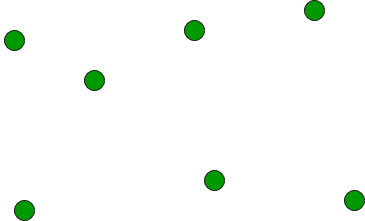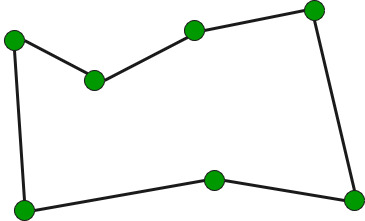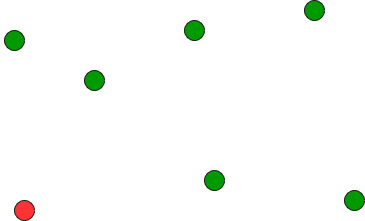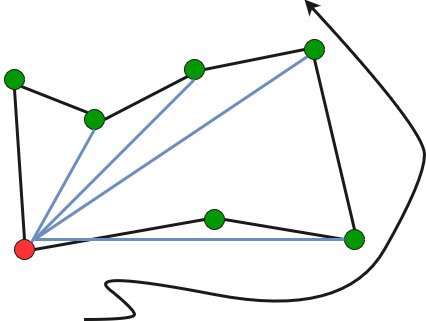GFG App
Open AppBrowser
Continue

# Find Simple Closed Path for a given set of points

Given a set of points, connect the dots without crossing.Example:

```Input: points[] = {(0, 3), (1, 1), (2, 2), (4, 4),
(0, 0), (1, 2), (3, 1}, {3, 3}};

Output: Connecting points in following order would
not cause any crossing
{(0, 0), (3, 1), (1, 1), (2, 2), (3, 3),
(4, 4), (1, 2), (0, 3)}```

We strongly recommend you to minimize your browser and try this yourself first.
The idea is to use sorting.

• Find the bottom-most point by comparing y coordinate of all points. If there are two points with same y value, then the point with smaller x coordinate value is considered. Put the bottom-most point at first position.• Consider the remaining n-1 points and sort them by polar angle in counterclockwise order around points. If polar angle of two points is same, then put the nearest point first.
• Traversing the sorted array (sorted in increasing order of angle) yields simple closed path.How to compute angles?
One solution is to use trigonometric functions.
Observation: We don’t care about the actual values of the angles. We just want to sort by angle.
Idea: Use the orientation to compare angles without actually computing them!

Below is C++ implementation of above idea.

## C++

 `// A C++ program to find simple closed path for n points` `// for explanation of orientation()` `#include ` `using` `namespace` `std;`   `struct` `Point` `{` `    ``int` `x, y;` `};`   `// A global point needed for  sorting points with reference` `// to the first point. Used in compare function of qsort()` `Point p0;`   `// A utility function to swap two points` `int` `swap(Point &p1, Point &p2)` `{` `    ``Point temp = p1;` `    ``p1 = p2;` `    ``p2 = temp;` `}`   `// A utility function to return square of distance between` `// p1 and p2` `int` `dist(Point p1, Point p2)` `{` `    ``return` `(p1.x - p2.x)*(p1.x - p2.x) +` `           ``(p1.y - p2.y)*(p1.y - p2.y);` `}`   `// To find orientation of ordered triplet (p, q, r).` `// The function returns following values` `// 0 --> p, q and r are collinear` `// 1 --> Clockwise` `// 2 --> Counterclockwise` `int` `orientation(Point p, Point q, Point r)` `{` `    ``int` `val = (q.y - p.y) * (r.x - q.x) -` `              ``(q.x - p.x) * (r.y - q.y);`   `    ``if` `(val == 0) ``return` `0;  ``// collinear` `    ``return` `(val > 0)? 1: 2; ``// clockwise or counterclock wise` `}`   `// A function used by library function qsort() to sort` `//  an array of points with respect to the first point` `int` `compare(``const` `void` `*vp1, ``const` `void` `*vp2)` `{` `   ``Point *p1 = (Point *)vp1;` `   ``Point *p2 = (Point *)vp2;`   `   ``// Find orientation` `   ``int` `o = orientation(p0, *p1, *p2);` `   ``if` `(o == 0)` `     ``return` `(dist(p0, *p2) >= dist(p0, *p1))? -1 : 1;`   `   ``return` `(o == 2)? -1: 1;` `}`   `// Prints simple closed path for a set of n points.` `void` `printClosedPath(Point points[], ``int` `n)` `{` `   ``// Find the bottommost point` `   ``int` `ymin = points.y, min = 0;` `   ``for` `(``int` `i = 1; i < n; i++)` `   ``{` `     ``int` `y = points[i].y;`   `     ``// Pick the bottom-most. In case of tie, choose the` `     ``// left most point` `     ``if` `((y < ymin) || (ymin == y &&` `         ``points[i].x < points[min].x))` `        ``ymin = points[i].y, min = i;` `   ``}`   `   ``// Place the bottom-most point at first position` `   ``swap(points, points[min]);`   `   ``// Sort n-1 points with respect to the first point.` `   ``// A point p1 comes before p2 in sorted output if p2` `   ``// has larger polar angle (in counterclockwise` `   ``// direction) than p1` `   ``p0 = points;` `   ``qsort``(&points, n-1, ``sizeof``(Point), compare);`   `   ``// Now stack has the output points, print contents` `   ``// of stack` `   ``for` `(``int` `i=0; i

## Java

 `import` `java.util.*;`   `class` `Point {` `    ``int` `x, y;` `    ``Point(``int` `x, ``int` `y) {` `        ``this``.x = x;` `        ``this``.y = y;` `    ``}` `}`   `class` `ConvexHull {` `    ``static` `Point p0;`   `    ``static` `void` `swap(Point p1, Point p2) {` `        ``Point temp = p1;` `        ``p1 = p2;` `        ``p2 = temp;` `    ``}`   `    ``static` `int` `dist(Point p1, Point p2) {` `        ``return` `(``int``)Math.pow(p1.x - p2.x, ``2``) +` `               ``(``int``)Math.pow(p1.y - p2.y, ``2``);` `    ``}`   `    ``static` `int` `orientation(Point p, Point q, Point r) {` `        ``int` `val = (q.y - p.y) * (r.x - q.x) -` `                  ``(q.x - p.x) * (r.y - q.y);`   `        ``if` `(val == ``0``) ``return` `0``; ``// collinear` `        ``return` `(val > ``0``)? ``1``: ``2``; ` `    ``}`   `    ``static` `int` `compare(Point p1, Point p2) {` `        ``int` `o = orientation(p0, p1, p2);` `        ``if` `(o == ``0``)` `            ``return` `(dist(p0, p2) >= dist(p0, p1))? -``1` `: ``1``;` `        ``return` `(o == ``2``)? -``1``: ``1``;` `    ``}`   `    ``static` `void` `printClosedPath(Point points[], ``int` `n) {` `        ``int` `ymin = points[``0``].y, min = ``0``;` `        ``for` `(``int` `i = ``1``; i < n; i++) {` `            ``int` `y = points[i].y;` `            ``if` `((y < ymin) || (ymin == y &&` `                ``points[i].x < points[min].x))` `                ``ymin = points[i].y;` `                ``min = i;` `        ``}` `        ``swap(points[``0``], points[min]);` `        ``p0 = points[``0``];` `        ``Arrays.sort(points, ``1``, n, (p1, p2) -> compare(p1, p2));` `        ``for` `(``int` `i=``0``; i

## Python3

 `from` `functools ``import` `cmp_to_key` `# A Python program to find simple closed path for n points` `# for explanation of orientation()`   `# A global point needed for  sorting points with reference` `# to the first point. Used in compare function of qsort()` `p0 ``=` `None`   `# A utility function to return square of distance between` `# p1 and p2` `def` `dist(p1, p2):` `    ``return` `(p1[``0``] ``-` `p2[``0``])``*``(p1[``0``] ``-` `p2[``0``]) ``+` `(p1[``1``] ``-` `p2[``1``])``*``(p1[``1``] ``-` `p2[``1``])`   `# To find orientation of ordered triplet (p, q, r).` `# The function returns following values` `# 0 --> p, q and r are collinear` `# 1 --> Clockwise` `# 2 --> Counterclockwise` `def` `orientation(p, q, r):` `    ``val ``=` `(q[``1``] ``-` `p[``1``]) ``*` `(r[``0``] ``-` `q[``0``]) ``-` `(q[``0``] ``-` `p[``0``]) ``*` `(r[``1``] ``-` `q[``1``])`   `    ``if` `val ``=``=` `0``: ``return` `0`  `# collinear` `    ``return` `1` `if` `val > ``0` `else` `2` `# clockwise or counterclock wise`   `# A function used by library function qsort() to sort` `#  an array of points with respect to the first point` `def` `compare(vp1, vp2):` `    ``p1 ``=` `vp1` `    ``p2 ``=` `vp2`   `    ``# Find orientation` `    ``o ``=` `orientation(p0, p1, p2)` `    ``if` `o ``=``=` `0``:` `        ``return` `-``1` `if` `dist(p0, p2) >``=` `dist(p0, p1) ``else` `1`   `    ``return` `-``1` `if` `o ``=``=` `2` `else` `1`   `# Prints simple closed path for a set of n points.` `def` `printClosedPath(points, n):` `    ``global` `p0` `    ``# Find the bottommost point` `    ``ymin ``=` `points[``0``][``1``]` `    ``min` `=` `0` `    ``for` `i ``in` `range``(``1``,n):` `        ``y ``=` `points[i][``1``]`   `        ``# Pick the bottom-most. In case of tie, choose the` `        ``# left most point` `        ``if` `(y < ymin) ``or` `(ymin ``=``=` `y ``and` `points[i][``0``] < points[``min``][``0``]):` `            ``ymin ``=` `points[i][``1``]` `            ``min` `=` `i`   `    ``# Place the bottom-most point at first position` `    ``temp ``=` `points[``0``]` `    ``points[``0``] ``=` `points[``min``]` `    ``points[``min``] ``=` `temp`   `    ``# Sort n-1 points with respect to the first point.` `    ``# A point p1 comes before p2 in sorted output if p2` `    ``# has larger polar angle (in counterclockwise` `    ``# direction) than p1` `    ``p0 ``=` `points[``0``]` `    ``points.sort(key``=``cmp_to_key(compare))`   `    ``# Now stack has the output points, print contents` `    ``# of stack` `    ``for` `i ``in` `range``(n):` `        ``print``(``"("``,points[i][``0``],``","``,points[i][``1``],``"), "``, end``=``"")`   `# Driver program to test above functions` `points ``=` `[[``0``, ``3``], [``1``, ``1``], [``2``, ``2``], [``4``, ``4``], [``0``, ``0``], [``1``, ``2``], [``3``, ``1``], [``3``, ``3``]]` `n ``=` `len``(points)`   `printClosedPath(points, n)`

## C#

 `using` `System;` `using` `System.Collections.Generic;`   `public` `class` `Point {` `    ``public` `int` `x, y;` `    ``public` `Point(``int` `x, ``int` `y)` `    ``{` `        ``this``.x = x;` `        ``this``.y = y;` `    ``}` `}`   `public` `class` `ClosestPath {` `    ``static` `Point p0;`   `    ``static` `int` `dist(Point p1, Point p2)` `    ``{` `        ``return` `(p1.x - p2.x) * (p1.x - p2.x)` `            ``+ (p1.y - p2.y) * (p1.y - p2.y);` `    ``}`   `    ``static` `int` `orientation(Point p, Point q, Point r)` `    ``{` `        ``int` `val = (q.y - p.y) * (r.x - q.x)` `                  ``- (q.x - p.x) * (r.y - q.y);` `        ``if` `(val == 0)` `            ``return` `0; ``// collinear` `        ``return` `(val > 0)` `            ``? 1` `            ``: 2; ``// clockwise or counterclockwise` `    ``}`   `    ``static` `int` `compare(Point p1, Point p2)` `    ``{` `        ``int` `o = orientation(p0, p1, p2);` `        ``if` `(o == 0)` `            ``return` `(dist(p0, p2) >= dist(p0, p1)) ? -1 : 1;` `        ``return` `(o == 2) ? -1 : 1;` `    ``}`   `    ``static` `void` `printClosedPath(List points, ``int` `n)` `    ``{` `        ``// Find the bottommost point` `        ``int` `ymin = points.y;` `        ``int` `min = 0;` `        ``for` `(``int` `i = 1; i < n; i++) {` `            ``int` `y = points[i].y;` `            ``if` `((y < ymin)` `                ``|| (ymin == y` `                    ``&& points[i].x < points[min].x)) {` `                ``ymin = points[i].y;` `                ``min = i;` `            ``}` `        ``}`   `        ``// Place the bottom-most point at first position` `        ``Point temp = points;` `        ``points = points[min];` `        ``points[min] = temp;`   `        ``// Sort n-1 points with respect to the first point.` `        ``// A point p1 comes before p2 in sorted output if p2` `        ``// has larger polar angle (in counterclockwise` `        ``// direction) than p1` `        ``p0 = points;` `        ``points.Sort(compare);`   `        ``// Now stack has the output points, print contents` `        ``// of stack` `        ``for` `(``int` `i = 0; i < n; i++) {` `            ``Console.Write(``"("` `+ points[i].x + ``", "` `                          ``+ points[i].y + ``"), "``);` `        ``}` `    ``}`   `    ``public` `static` `void` `Main()` `    ``{` `        ``List points = ``new` `List() {` `            ``new` `Point(0, 3), ``new` `Point(1, 1),` `                ``new` `Point(2, 2), ``new` `Point(4, 4),` `                ``new` `Point(0, 0), ``new` `Point(1, 2),` `                ``new` `Point(3, 1), ``new` `Point(3, 3)` `        ``};` `        ``int` `n = points.Count;`   `        ``printClosedPath(points, n);` `    ``}` `}` `// This code is contributed by user_dtewbxkn77n`

## Javascript

 `// A javascript program to find simple closed path for n points` `// for explanation of orientation()`   `// A global point needed for  sorting points with reference` `// to the first point. Used in compare function of qsort()` `let p0;`   `// A utility function to return square of distance between` `// p1 and p2` `function` `dist(p1, p2)` `{` `    ``return` `(p1 - p2)*(p1 - p2) +` `           ``(p1 - p2)*(p1 - p2);` `}`   `// To find orientation of ordered triplet (p, q, r).` `// The function returns following values` `// 0 --> p, q and r are collinear` `// 1 --> Clockwise` `// 2 --> Counterclockwise` `function` `orientation(p, q, r)` `{` `    ``let val = (q - p) * (r - q) - (q - p) * (r - q);`   `    ``if` `(val == 0) ``return` `0;  ``// collinear` `    ``return` `(val > 0)? 1: 2; ``// clockwise or counterclock wise` `}`   `// A function used by library function qsort() to sort` `//  an array of points with respect to the first point` `function` `compare(vp1, vp2)` `{` `   ``let p1 = vp1;` `   ``let p2 = vp2;`   `   ``// Find orientation` `   ``let o = orientation(p0, p1, p2);` `   ``if` `(o == 0)` `     ``return` `(dist(p0, p2) >= dist(p0, p1))? -1 : 1;`   `   ``return` `(o == 2)? -1: 1;` `}`   `// Prints simple closed path for a set of n points.` `function` `printClosedPath(points, n)` `{` `   ``// Find the bottommost point` `   ``let ymin = points;` `   ``let min = 0;` `   ``for` `(let i = 1; i < n; i++)` `   ``{` `     ``let y = points[i];` `     `  `     ``// Pick the bottom-most. In case of tie, choose the` `     ``// left most point` `     ``if` `((y < ymin) || (ymin == y && points[i] < points[min])){` `        ``ymin = points[i];` `        ``min = i;` `     ``}`   `   ``}`   `   ``// Place the bottom-most point at first position` `   ``let temp = points;` `    ``points = points[min];` `    ``points[min] = temp;`   `   ``// Sort n-1 points with respect to the first point.` `   ``// A point p1 comes before p2 in sorted output if p2` `   ``// has larger polar angle (in counterclockwise` `   ``// direction) than p1` `   ``p0 = points;` `   ``points.sort(compare);`   `   ``// Now stack has the output points, print contents` `   ``// of stack` `   ``for` `(let i=0; i

Output:

```(0, 0), (3, 1), (1, 1), (2, 2), (3, 3),
(4, 4), (1, 2), (0, 3), ```

Time complexity of above solution is O(n Log n) if we use a O(nLogn) sorting algorithm for sorting points.
Auxiliary Space: O(1), since no extra space has been taken.

Source:
http://www.dcs.gla.ac.uk/~pat/52233/slides/Geometry1x1.pdf